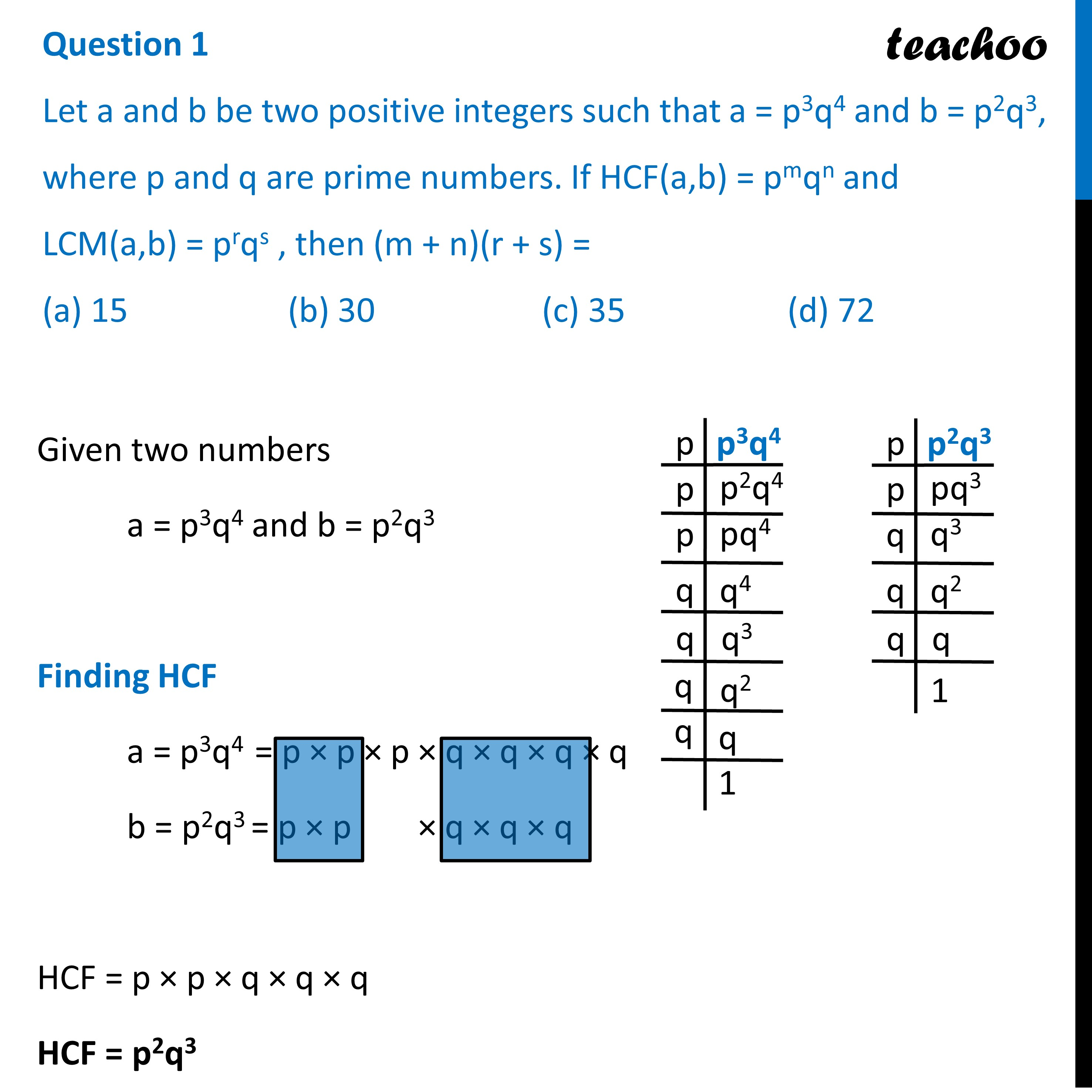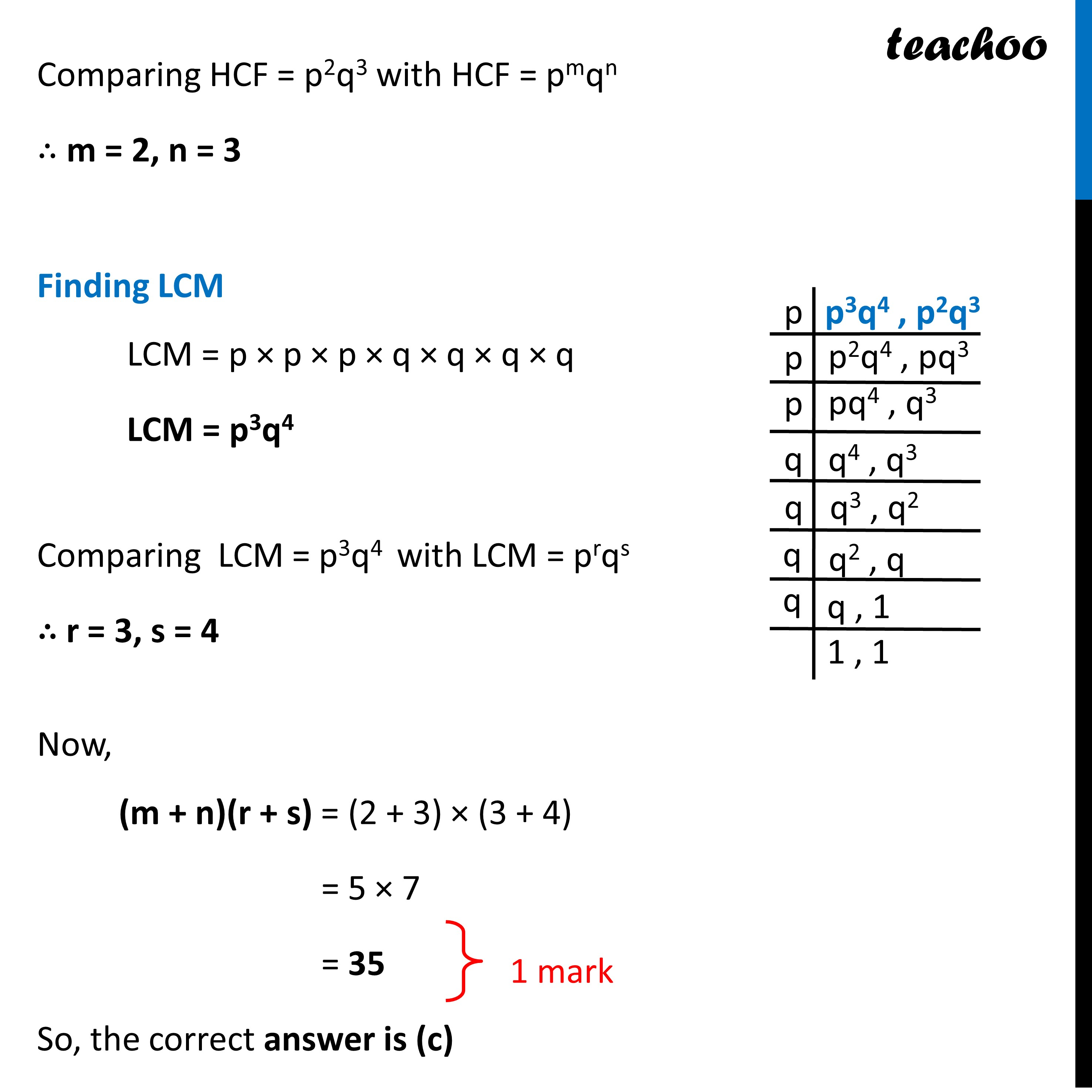CBSE Class 10 Sample Paper for 2023 Boards - Maths Standard

Class 10
Solutions of Sample Papers for Class 10 Boards

## (a) 15   (b) 30  (c) 35  (d) 72

This question is similar to Question 7 - CBSE Class 10 Sample Paper for 2018 BoardsLearn in your speed, with individual attention - Teachoo Maths 1-on-1 Class

### Transcript

Question 1 Let a and b be two positive integers such that a = p3q4 and b = p2q3, where p and q are prime numbers. If HCF(a,b) = pmqn and LCM(a,b) = prqs , then (m + n)(r + s) = (a) 15 (b) 30 (c) 35 (d) 72 Given two numbers a = p3q4 and b = p2q3 Finding HCF a = p3q4 = p × p × p × q × q × q × q b = p2q3 = p × p × q × q × q HCF = p × p × q × q × q HCF = p2q3 Comparing HCF = p2q3 with HCF = pmqn ∴ m = 2, n = 3 Finding LCM LCM = p × p × p × q × q × q × q LCM = p3q4 Comparing LCM = p3q4 with LCM = prqs ∴ r = 3, s = 4 Now, (m + n)(r + s) = (2 + 3) × (3 + 4) = 5 × 7 = 35 So, the correct answer is (c)